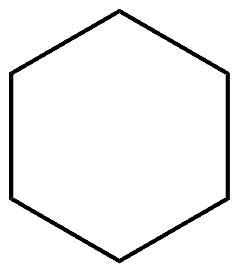# Torsional Angle

## Torsional Angle Definition:

The Tortional angle is the angle by which two groups are separated in a Newman projection. It is also called the dihedral angle.

## Torsional Angle Explained:

The angle that is between two intersecting planes, through two sets of three atoms, having two atoms that are similar. See Dihedral angle.

×

Cart